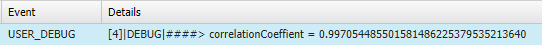## Excel FISHER Function in Apex Salesforce

In this post we will see how we can write Excel FISHER function in Apex Salesforce. Before going to the apex code lets first see some details about FISHER function. This function returns the fisher transformation at x.

Syntax of FISHER function is as follows in Excel

• FISHER(x)
• WHERE x is required
• Value of x should be greater than -1 and less than 1 i.e -1 < x < 1.

Now see the code for Excel FISHER function in Apex Salesforce.

Above code can be added in any apex class, after adding this code we can use it as per our requirement. Lets test it from developer console. Go to developer console and in Execute anonymous window paste the below code.

After executing above code snippet you will get below output.

Hope this code will save your time, if you need to implement FISHER function in salesforce.

Happy Coding 🙂
799 The Coder

See some more post for Excel function in Apex

## Excel CORREL function in Apex Salesforce

Today we will see salesforce apex equivalent code for Excel CORREL function. Excel CORREL function in Apex Salesforce can be used if we need to perform same calculation on salesforce data as excel CORREL  function.

Syntax of CORREL function is as follows in Excel

• CORREL(array1, array2)
• WHERE array1 and array2 both are Required.
• We must ensure that array1 and array2 are same in size.

See the code below, code has static methods written you can put them in your util class/helper class.

Once we have above methods in our code, we can use it 🙂 , Now lets test the method from developer console, open developer console and write below code.

When you execute the above code, you will get the output as follows.correlationCoeffient = 0.997054485501581486225379535213640

See more Excel functions in apex salesforce code

Happy Coding !

799 The Coder

## Excel INTERCEPT function in Apex Salesforce

Hello awesome Developers!!! This post will save your time if you are going to implement Excel INTERCEPT function in apex salesforce. In my previous post of excel function (Excel LINEST function in Apex Salesforce), we coded for LINEST function of excel.

Before we jump to code, lets have a quick view of INTERCEPT function.

Syntax of Intercept function is as follows in Excel

INTERCEPT(known_y’s, known_x’s)

WHERE Known_y’s  and Known_x’s  both are Required.

We must ensure that Known_y’s and Known_x’s size is same.

The above code snippet is a static method, you can put it any helper/utility class in salesforce.  See below a demo call to this method from developer console.

The output of the above code snippet from developer console will be below

INTERCEPT = 0.0483870967741935483870967741935484

Use it and save your time, in your saved time do some other creative coding 🙂 !!!